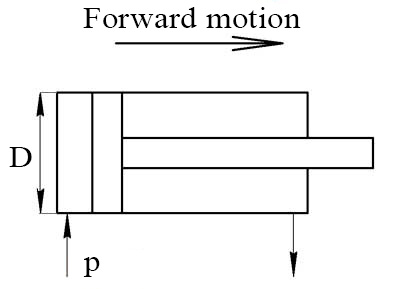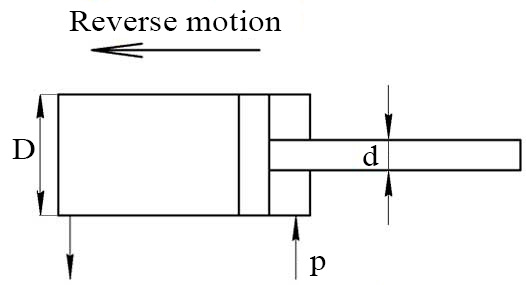# Hydraulic cylinder force calculator

The cylinder force F is dependent on the operating pressure p and the effective area A.

F = p×A

## The force produced on the opposite of rod side

The calculated equation:

F = p×πD2/4Pressure p: Units Pa Pascal kPa KiloPascal MPa MegaPascal Bar Bar psi Pound-force per square inch at Technical atmosphere Piston diameter D: Units mm Millimetre cm Centimeter dm Decimeter m Meter km Kilometer in Inch

## The force produced on the rod side

Equation for calculating the force of the double action hydraulic cylinder in the reverse motion:

F = p×π(D2-d2)/4Pressure p: Units Pa Pascal kPa KiloPascal MPa MegaPascal Bar Bar psi Pound-force per square inch at Technical atmosphere Piston diameter D: Rod diameter d: Units mm Millimetre cm Centimeter dm Decimeter m Meter km Kilometer in Inch

Languages
Spanish (ES) Deutsch (DE)
Featured articles
How to read hydraulic circuits
Hydraulic cylinders
Hydraulic accumulators
Best calculators
Pressure loss calculator
Calculating the system curve
Hydraulic cylinder force calculator
My Youtube channel
This site uses cookies to store information on your computer. Details